#Linear Second Order Differential EquationsA linear second order differential equations is written as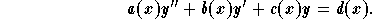When d(x) = 0, the equation is called homogeneous, otherwise it is called nonhomogeneous. To a nonhomogeneous equation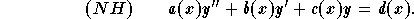,

we associate the so called associated homogeneous equation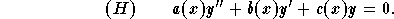For the study of these equations we consider the explicit ones given by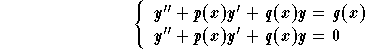where p(x) = b(x)/a(x), q(x) = c(x)/a(x) and g(x) = d(x)/a(x). If p(x), q(x) and g(x) are defined and continuous on the interval I, then the IVP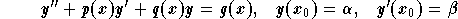,

where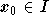and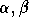are arbitrary numbers, has a unique solution defined on I.

Main result: The general solution to the equation (NH) is given by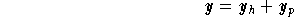,

where

(i)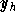is the general solution to the homogeneous associated equation (H);
(ii)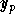is a particular solution to the equation (NH).

In conclusion, we deduce that in order to solve the nonhomogeneous equation (NH), we need to

Step 1: find the general solution to the homogeneous associated equation (H), say;
Step 2: find a particular solution to the equation (NH), say;
Step 3: write down the general solution to (NH) as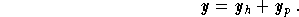[Differential Equations] [First Order D.E.] [Second Order D.E.]
[Geometry] [Algebra] [Trigonometry ]
[Calculus] [Complex Variables] [Matrix Algebra]S.O.S MATHematics home page

Do you need more help? Please post your question on our S.O.S. Mathematics CyberBoard.Author: Mohamed Amine Khamsi